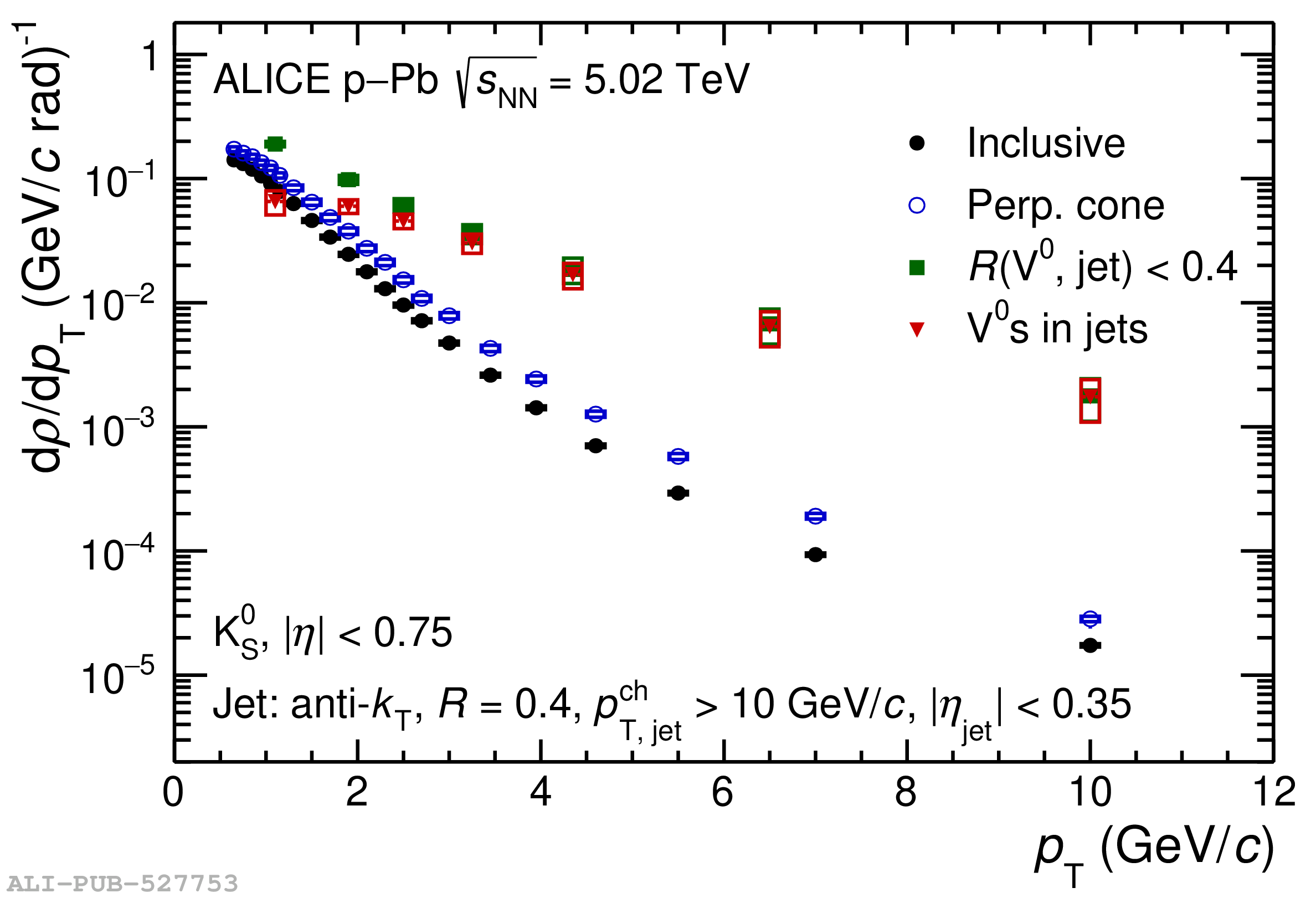# Figure 2

 The $\pT$-differential density of particles $\drhoVdpT$ (see Eq. \eqref{eq:defv0rho}) in \pPb collisions at \fivenn for \kzero\ (upper panel) and the sum of \lmb\ and \almb\ (lower panel) The density is shown for three selection criteria: inclusive particles from minimum bias events (black full circle), particles associated with the underlying event production estimated with PC selection (blue open circle, labelled as Perp. cone" in the figure), JC $\Vzero$s with $\Rvj < 0.4$ (green full square) The density distribution of $\Vzero$s in jets with UE background subtracted (defined by Eq. (\ref{eq:defJEV0s})) is shown as the red full triangle Statistical uncertainties and systematic uncertainties are shown as vertical bars and open boxes, respectively.